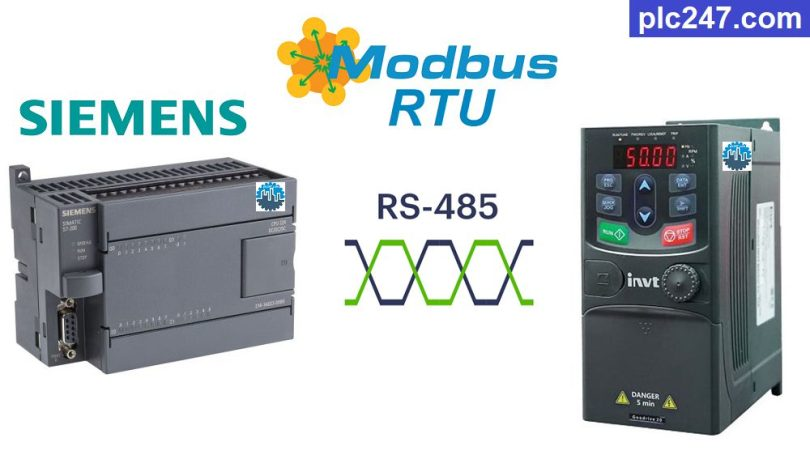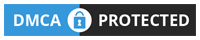# Siemens S7-200 “Modbus RTU” INVT GD20 InverterWritten by

GD20 inverter is a popular inverter series of INVT that controls light to medium loads with power from 0.4kW to 110kW. Products are widely used in textile machinery, food machinery, printing and packaging, environmental protection equipment, equipment, woodworking equipment, transportation equipment and other industries.

In particular, INVT Goodrive 20 also has an RS485 port integrated to make it controllable via Modbus-RTU communication protocol. Today plc247.com will guide you to control it by this protocol by using PLC Siemens S7-200.

#### Siemens S7-200 & INVT GD20 “Connection Diagram”

Below is a diagram of Modbus RS384 communication connection between Siemens S7-200 and INVT Goodrive 20 inverter:

#### INVT GD20 “Modbus RTU” Parameters Setting

Below are the communication parameters that need to be installed for the INVT GD20 inverter to be able to control it using the Modbus-RTU protocol:

+ P00.01 = “2” (Frequency Reference via RS485)

+ P00.06 = “8” (Motor Control via RS485)

+ P00.09 = “0” (“A Frequency” Selection)

+ P14.00 = “1” (Slave Address = 1)

+ P14.01 = “3” (9600bps)

+ P14.02 = “1” (Modbus-RTU 8bit/Even/stop bit = 1)

+ P14.03 = “5”

+ P14.04 = “0.0”

##### Note: Siemens S7-200 Holding Registers Address = “VFD Address (Dec) + 40001/400001”

>>> VFD Control Address = 2000(hex) + 40001 = 8192(dec)+ 40001 = 48193 (dec)

• Set Word “48193” = 1 >>> Forward Run
• Set Word “48193” = 2 >>> Reverse Run
• Set Word “48193” = 5 >>> Reverse Run

>>> Frequency Set Address = 2001(hex) + 40001 = 8193(dec)+ 40001 = 48194 (dec)

• Output Frequency Address = 3000 (hex) = 12288 (dec) + 400001 = 412289 (dec)
• Output Voltage Address = 3003 (hex) = 12291 (dec) + 400001 = 412292 (dec)
• Output Current Address = 3004 (hex) = 12292 (dec) + 400001 = 412293 (dec)

### PLC Programming Example

+ Communication Distribution

+ Port.0 Modbus Configuration

+ Frequency Setting Command

+ VFD Start/Stop Control

+ Data Monitoring

### Project Video Tutorial

======

###### References